#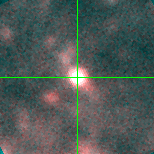M33C-15731 (J013350.12+304126.6)

## Target Notes### Classification: B[e]sg

One LGGS star less than 2 arcseconds away. Influence of unresolved blend is negligible in B (about 4 mags fainter). Earns 'b' for being unresolved in V (about 3 mags fainter) for being about size of error bar. Earns 'B' mark in both R ( about 2 mags fainter) and I (about 1 mag fainter) for influence greater than the size of the error bar. **Updated 180529***

## Plots of Brightness and Color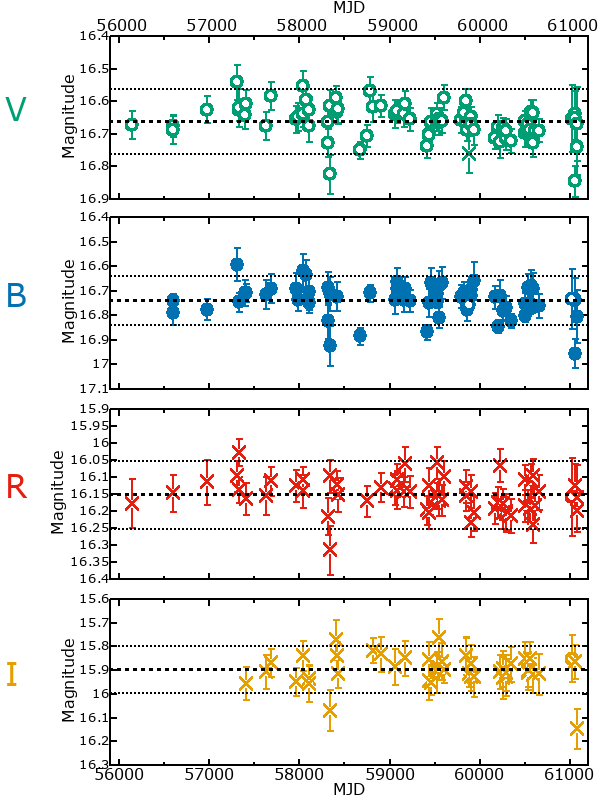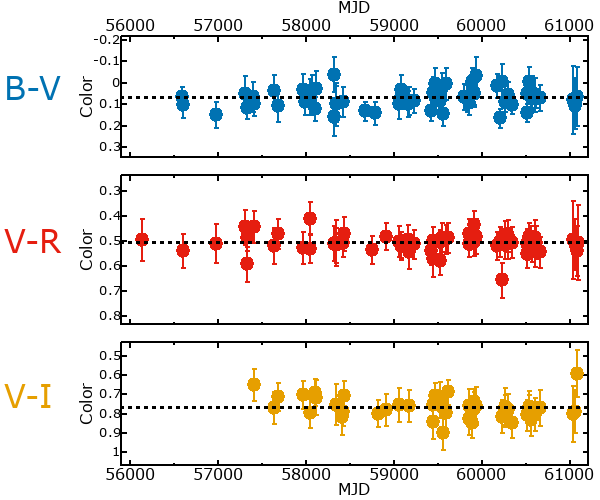## Variability

From the data available in this survey, it is not possible to tell with certainty if this star is variable.

### Correlation factors between bands

If the brightness variation is true variability then there should be a correlation between brightness changes recorded in different filter bands. The following table are the R-squared linear correlation factors calculated for the pairs of bands. The number in parentheses next to the R-squared value is the number of elements used to calculate the correlation factor. The number in square brackets next to the band is the range of magnitudes measured.

V [0.26 mag]B [0.27 mag]R [0.27 mag]
B [0.26 mag]0.58 (28)
R [0.27 mag]0.62 (25)0.46 (19)
I [0.22 mag]0.39 (15)0.57 (10)0.72 (11)

### Magnitude-Magnitude Plots

The plots below are provided to check the values in the table above and also to show any non-linear correlations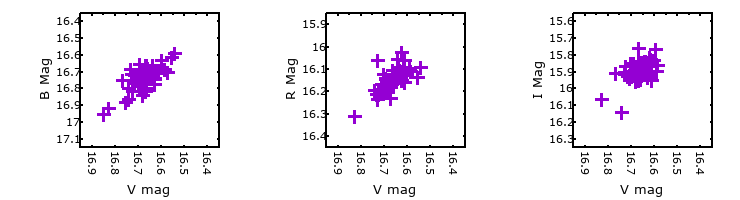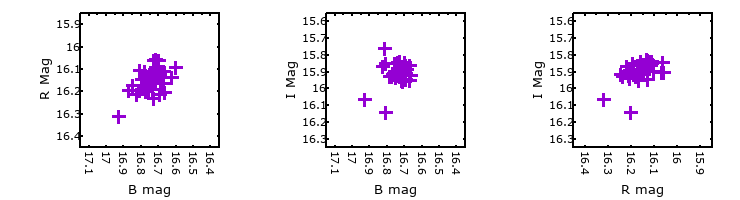### I & R Statistics

Welch & Stetson (1993, AJ, 105, 1813) present a method for varible star detection in CCD images from automated surveys. Data from two bands are compared and two statistics are calculated: R and I.

The I-statistic has an expectation value of zero for stars that are NOT variable. A larger non-zero I-value indicates a higher likelihood that the star is variable. The R statistic indicates how the color of the star changes as the variable grows brighter with R = 1.0 being no change in color.

R and I values have only been calculated if there are more than four (4) matched measurements of brightness in each band.
V
(I|R)
B
(I|R)
R
(I|R)
B
(I|R)
1.08 | 0.97
R
(I|R)
0.64 | 1.381.93 | 0.06
I
(I|R)
0.37 | 0.880.43 | 0.830.66 | 1.07